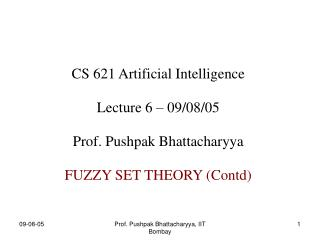DownloadDownload PresentationCS 621 Artificial Intelligence Lecture 6 – 09/08/05 Prof. Pushpak Bhattacharyya

# CS 621 Artificial Intelligence Lecture 6 – 09/08/05 Prof. Pushpak Bhattacharyya

Download Presentation## CS 621 Artificial Intelligence Lecture 6 – 09/08/05 Prof. Pushpak Bhattacharyya

- - - - - - - - - - - - - - - - - - - - - - - - - - - E N D - - - - - - - - - - - - - - - - - - - - - - - - - - -
##### Presentation Transcript

1. CS 621 Artificial Intelligence Lecture 6 – 09/08/05 Prof. Pushpak Bhattacharyya FUZZY SET THEORY (Contd) Prof. Pushpak Bhattacharyya, IIT Bombay

2. S Path 1 both optimal Path 2 G A1*, A2* A1* is less informed h1(n) < h2(n) n Digression Recap Prof. Pushpak Bhattacharyya, IIT Bombay

3. S 7 6 5 A1* h1(n) = 2, n A2* h2(n) = 3, n A B C 3 4 5 G Example Prof. Pushpak Bhattacharyya, IIT Bombay

4. A2* --> 1. OL: S CL: Φ 2. OL: A B C (fA=10, fB=9, fC=8) CL: S 3. OL: A(10), B(9), g(f=10) CL: S C 4. OL: A G CL: S C B 5. OL: A CL: S C B G Status of Lists in A2* Prof. Pushpak Bhattacharyya, IIT Bombay

5. A1* --> 1. OL: S CL: Φ 2. OL: A B C (fA=9, fB=9, fC=7) CL: S 3. OL: A(9), B(8), g(f=10) CL: S C Status of Lists in A1* Prof. Pushpak Bhattacharyya, IIT Bombay

6. Membership Predicate : 0 <= µS(x) <= 1 Profile : 2 dimension figure of µand its value Hedge : deals with adverb Linguistic Variable Definitions Seen Till Now Prof. Pushpak Bhattacharyya, IIT Bombay

7. Union : A, B : fuzzy sets µAυB(x) = max(µA(x), µB(x)) Intersection : µA∩B(x) = min(µA(x), µB(x)) Complementation : µAc(x) = 1 - µA(x) Fuzzy Set Operations Prof. Pushpak Bhattacharyya, IIT Bombay

8. 1. (Ac)c = A µ(Ac)c (x) = 1 - µAc (x) = 1 – (1 - µA(x)) = µA(x) QED 2. A υ Ac = Acυ A 3. A ∩ Ac = Ac∩ A 4. De Morgan (A υB)c = Ac∩ Bc (A ∩B)c = AcυBc Laws of Crisp Set Theory Apply Prof. Pushpak Bhattacharyya, IIT Bombay

9. 5. Associativity A υB υC = (A υB) υC = A υ(B υC) A ∩B ∩C = (A ∩B) ∩C = A ∩(B ∩C) 6. Distributivity A ∩(B υC) = (A ∩B) υ(A ∩C) More Laws Prof. Pushpak Bhattacharyya, IIT Bombay

10. Prove (A ∩B)c = AcυBc LHS: = µ(A∩B)c(x) = 1 - µ(A∩B)(x) = 1 - min(µA(x) , µB(x)) = max (1 - µA(x) , 1 - µB(x)) = RHS Proof Prof. Pushpak Bhattacharyya, IIT Bombay

11. X = {x1,x2} UNIVERSE CRISP SUBSETS of X are Φ, {x1}, {x2}, {x1, x2} (1,1) x2 (0,1) UNIT SQUARE x1 (0,0) (1,0) Representation of Fuzzy Sets • Sets can be represented by membership values. • Given n-element UNIVERSE, the subsets of the UNIVERSE correspond to the CORNERS of the HYPERCUBE which is UNIT • Fuzzy subsets of U are the points within the UNIT HYPERCUBE • Infinite number of fuzzy subsets Prof. Pushpak Bhattacharyya, IIT Bombay

12. A fuzzy set S is represented by S = {µS(x1)/x1, µS(x2)/x2 , .... , µS(xn)/xn} (1,1) {x2} (0,1) {x1, x2} A = {.3/x1, .5/x2} Φ {x1} x1 (0,0) (1,0) Representation (Contd) A(.3, .5) Ac Prof. Pushpak Bhattacharyya, IIT Bombay

13. (1,1) {x2} (0,1) {x1, x2} Corners : A, Ac, A∩Ac, AυAc Φ {x1} x1 (0,0) (1,0) Unit Square AυAc A A∩Ac Ac Fuzziness decreases as we move towards the corners. At the centre, fuzziness is maximum. Prof. Pushpak Bhattacharyya, IIT Bombay

14. Unit Square (Contd) Centre corresponds to “Russel's Paradox” Statement: A barber in a city shaves only and all those who do not shave themselves. Ques: Does the barber shave himself? P → Barber shaves himself P → ~P also ~P → P so, P ≡ ~P t(P) = 1 – t(P) so, t(P) = 0.5 Prof. Pushpak Bhattacharyya, IIT Bombay

15. A point nearer to the corner is less fuzzy. Measure of fuzziness = Entropy of fuzzy set Entropy = E(A) = d(A, Anear) / d(A, Afar) Anear = point closest to A out of the 4 corners Afar = farthest corner point Degree of Fuzziness Prof. Pushpak Bhattacharyya, IIT Bombay

16. d = distance between A, B = ∑x |µA(x) - µB(x) | A = {0.3/x1, 0.7/x2} B = {0.8/x1, 0.1/x2} d(A,B) = 0.5 + 0.6 = 1.1 L1 - distance Degree (Contd) Prof. Pushpak Bhattacharyya, IIT Bombay

17. (1,1) x2 (0,1) {x1, x2} x1 (0,0) (1,0) Entropy E(Acentre) = 1 0 <= E(A) <= 1 A B Theorem: E(A) = m(A ∩ Ac) / m(A υAc) m(A) = “cardinality” of A = Σx µA(x) Prof. Pushpak Bhattacharyya, IIT Bombay

18. Proof Sketch From definition E(A) = d(A, Anear) / d(A, Afar) d(A, Anear) = ∑xi| µA(xi) - µA near(xi) | d(A, Afar) = ∑xi| µA(xi) - µA far(xi) | m(A Ac) = ∑xi min(µA(xi), µAc (xi)) m(A Ac) = ∑ximax(µA(xi), µAc (xi)) Prof. Pushpak Bhattacharyya, IIT Bombay

19. Fuzziness & Probability Both model uncertainity. Sum of membership values ≠ 1 necessarily. Entropy(A), relates to probability of an event. Prof. Pushpak Bhattacharyya, IIT Bombay

20. Summary • Fuzzy set operations: generalizations of crisp set operations • Geometric representation of fuzzy set • Introduced degree of fuzziness • Measured in terms of entropy. Prof. Pushpak Bhattacharyya, IIT Bombay